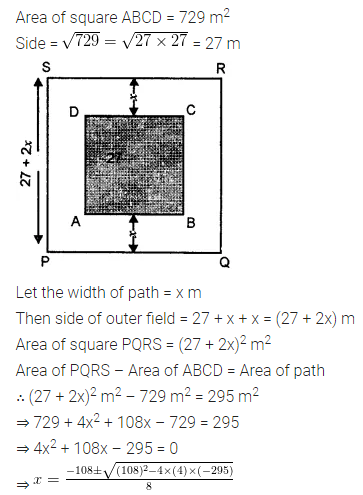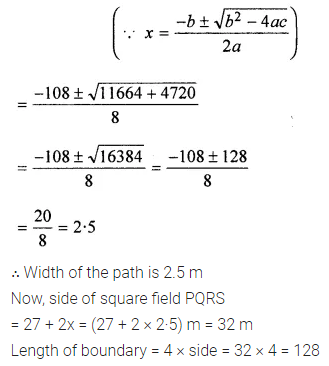## Online Education ML Aggarwal Class 8 Solutions for ICSE Maths Chapter 18 Mensuration Ex 18.1

Question 1.
The length and breadth of a rectangular field are in the ratio 9 : 5. If the area of the field is 14580 square metres, find the cost of surrounding the field with a fence at the rate of ₹3·25 per metre.
Solution: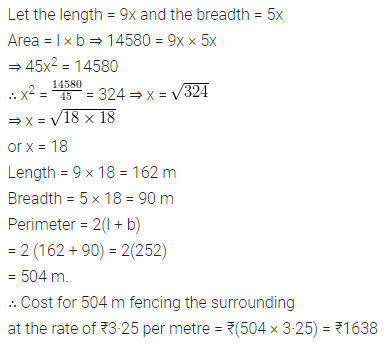Question 2.
A rectangle is 16 m by 9 m. Find a side of the square whose area equals the area of the rectangle. By how much does the perimeter of the rectangle exceed the perimeter of the square?
Solution: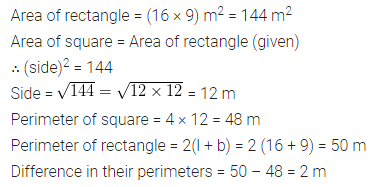Question 3.
Two adjacent sides of a parallelogram are 24 cm and 18 cm. If the distance between longer sides is 12 cm, find the distance between shorter sides.
Solution:Question 4.
Rajesh has a square plot with the measurement as shown in the given figure. He wants to construct a house in the middle of the plot. A garden is developed around the house. Find the total cost of developing a garden around the house at the rate of ₹50 per m2.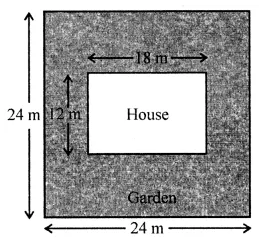Solution:Question 5.
A flooring tile has a shape of a parallelogram whose base is 18 cm and the corresponding height is 6 cm. How many such tiles are required to cover a floor of area 540 m2? (If required you can split the tiles in whatever way you want to fill up the comers).
Solution:Question 6.
An ant is moving around a few food pieces of different shapes scattered on the floor. For which food piece would the ant have to take a longer round?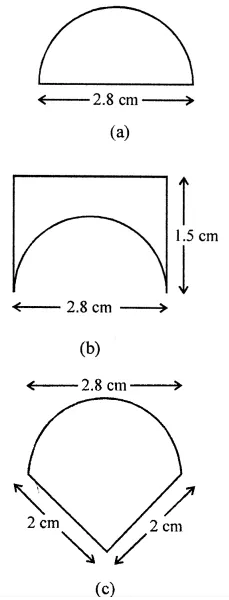Solution:Question 7.
In the adjoining figure, the area enclosed between the concentric circles is 770 cm2. If the radius of the outer circle is 21 cm, calculate the radius of the inner circle.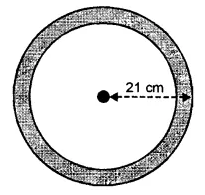Solution: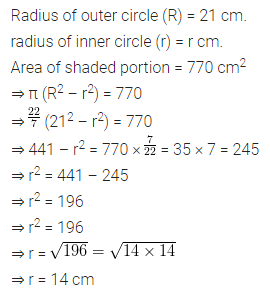Question 8.
A copper wire when bent in the form of a square encloses an area of 121 cm2. If the same wire is bent into the form of a circle, find the area of the circle.
Solution: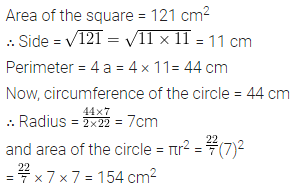Question 9.
From the given figure, find
(i) the area of ∆ ABC
(ii) length of BC
(iii) the length of altitude from A to BCSolution:Question 10.
A rectangular garden 80 m by 40 m is divided into four equal parts by two cross-paths 2.5 m wide. Find
(i) the area of the cross-paths.
(ii) the area of the unshaded portion.Solution: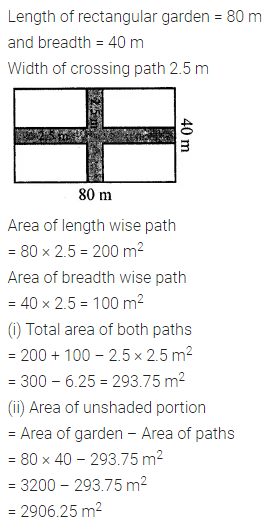Question 11.
In the given figure, ABCD is a rectangle. Find the area of the shaded region.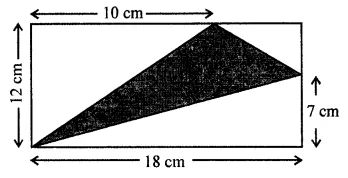Solution: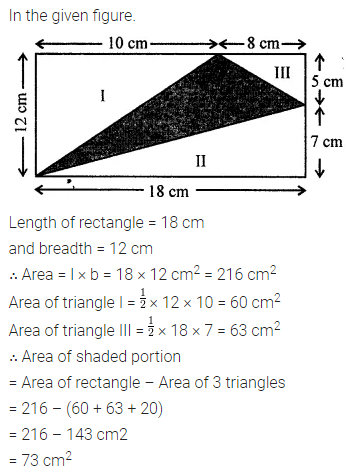Question 12.
In the adjoining figure, ABCD is a square grassy lawn of area 729 m2. A path of uniform width runs all around it. If the area of the path is 295 m2, find
(i) the length of the boundary of the square field enclosing the lawn and the path.
(ii) the width of the path.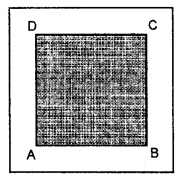Solution: